## Abstract

Flow-induced loads and Strouhal frequencies of secondary hyperboloid reflector which is located at top of the central tower of a proposed solar power plant are investigated. Initially for validation, flow-induced vibration characteristics of 3-D rectangular cylinder are studied and found in good agreement with previously published results. After the validation of the present numerical procedure, the force coefficients and the Strouhal frequencies of solar secondary hyperboloid reflector are determined for operational and survival wind speeds. Influences of various numerical parameters are investigated through the statistical values of the drag and the lift coefficients, Strouhal number, and pressure distribution. Finite element analysis has also been done to find out the deflections and stresses due to dead weight, imposed loads and wind loads and to optimize the design of hyperboloid and towers.

## Keywords

Hyperboloid reflector; CFD; Wind loads; Strouhal frequency; Structural analysis

## 1. Introduction

Nowadays, the great verity of solar technologies for electricity generation is available and among many, the application of reflector in large sizes is employed in many engineering systems. The amount of solar radiation entering the aperture of a collector depends on the local solar energy potential. Application of reflectors for solar heating and solar power plant improved in the recent years. Most of the solar power plants installed with reflectors are on flat terrain and they may be subjected to some environmental problems. One of the problems for such a large reflector is their stability to track the sun very accurately . Solar thermal power plants are a gifted alternative to cover the significant parts of growing energy demand. Fig. 1 shows the schematic diagram of typical molten salt type solar power plant. In this concept, the central tower has a secondary hyperboloid mirror surrounded by heliostats. The sunrays from all the heliostats are reflected downward by hyperboloid to a ground based receiver which absorbs the solar radiation and transfers to a molten salt steam generating system. The steam is then used to run a turbine generator system as in a conventional power plant. Concentrating thermal solar power has already become an established large-scale solar power technology, though its cost is not yet modest with conventional alternatives; therefore, considerable cost reductions as a significance of technology knowledge and large mass production are still essential .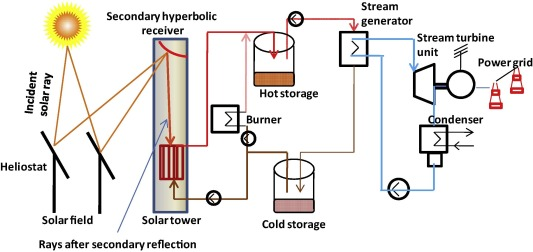Figure 1. Solar power plant.

Wind forces play a major role in design and operation of large reflectors and need for satisfactory estimates of these forces is becoming increasingly evident . Studies of wind loads on structure and boundary layer over different bodies such as buildings , hills, towers , arch roof, automobiles, heliostat , parachute, and dish are extensive both experimentally and theoretically. Several numerical and experimental studies have been performed to assess the thermal loading and heat transfer characteristics of large paraboloidal solar dish  and . Conversely, wind flow around parabolic and hyperbolic shapes is rare. In engineering, fluid forces and Strouhal numbers are the primary factors considered in the design of structures subjected to cross flow, e.g., chimney stacks, tube bundles in heat exchangers, overhead power cables, bridge piers, stays, chemical reaction towers, power plant towers, offshore platforms and adjacent skyscrapers.

Very few literatures are attempting flow around the 3-D bluff bodies  and . However, to the authors’ knowledge, most of the 3-D numerical simulations were carried out at low Reynolds numbers, i.e. Re < 103. However, for real case applications, e.g. high wind load on dishes, offshore structures at sea and vehicles in the air, the Reynolds number can easily reach high values, e.g. Re = 105–108. Thus, 3-D numerical simulations of flow around a hyperboloid reflector at high Reynolds numbers are required. In this study, the 3-D flow past a hyperboloid reflector around Re = 107 is investigated using a large-eddy simulation (LES) method. In this paper, numerical simulation is carried out through Open Source Field Operation and Manipulation () which has been available as an open-source code.

### 1.1. Need for study

In this work, a hyperbolic reflector, which is little more than quarter segment of full hyperboloid, is taken for analysis. IS 875 Part 3  provides force coefficient for most structural shapes. This coefficient when multiplied by design wind pressure and effective area of structure provides wind load on structures . Since IS 875 Part 3  does not provide force coefficient for hyperboloid surface, CFD analysis has been performed to find out the forces due to wind loads on hyperboloid surface. Shedding frequencies have also been obtained by CFD analysis.

### 1.2. Present objective

The objectives of this study were to compute the flow-induced loads and Strouhal frequencies of secondary hyperboloid reflector which is located at top of the central tower and to perform the structural integrity analysis of the central tower of a proposed solar power plant against wind load. The objectives of the present works are,

• To compute the wind load on secondary hyperboloid reflector for operational (40 km/h) and survival (160 km/h) wind speeds through 3-D LES simulation.
• To find the Strouhal frequencies of solar secondary hyperboloid reflector for operational (40 km/h) and survival (160 km/h) wind speeds.
• To ensure the safety of the central tower by keeping structural frequency away from shedding frequency of the wind to avoid the lock-in effect and also to check stresses are within allowable limits.

## 2. Numerical methodology

### 2.1. Governing equations

A Large Eddy Simulation (LES) is a simulation model in which the large eddies are computed and the smallest eddies are modeled with a subgrid scale (SGS) model in order to represent the effects of unresolved motions on the resolved scales. The major fact is that the largest eddies are directly affected by the boundary conditions and must be computed. While comparing with DNS, one can take larger time steps, since LES only involves modeling of the smallest eddies. Here, a filtering is introduced to decompose the variable ${\textstyle \phi }$ into the sum of a filtered component, ${\textstyle {\overline {\phi }}}$ and a subgrid scale component, ${\textstyle {\phi }^{'}}$. By applying the filtering operation to the NS equations for an incompressible flow, the filtered NS equations can be written as,

 ${\frac {\partial ({\overline {u}}_{i})}{\partial x_{i}}}=0{\mbox{,}}$
(1)

 ${\frac {\partial ({\overline {u}}_{i})}{\partial t}}+{\frac {\partial ({\overline {u}}_{i}{\overline {u}}_{j})}{\partial x_{j}}}=$$-{\frac {1}{\rho }}{\frac {\partial {\overline {p}}}{\partial x_{i}}}+$$\upsilon {\frac {{\partial }^{2}({\overline {u}}_{i})}{\partial x_{i}x_{j}}}-$${\frac {\partial {\tau }_{ij}}{\partial x_{j}}}{\mbox{,}}$
(2)

where i, = 1–3; and ${\textstyle {\overline {u}}_{1}{\mbox{,}}\quad {\overline {u}}_{2}}$ and ${\textstyle {\overline {u}}_{3}}$ denote resolved velocity components in three directions. ${\textstyle \rho }$ and ${\textstyle {\overline {p}}}$ are the fluid density and resolved pressure respectively; and t   is the time. The subgrid stress ${\textstyle \rho }$ is given by,

 ${\tau }_{ij}={\overline {u_{i}u_{j}}}-{\overline {u}}_{i}{\overline {u}}_{j}{\mbox{.}}$
(3)

In order to solve Eqs. (1) and (2), the ${\textstyle {\tau }_{ij}}$ is need to be modeled. Smagorinsky  was the first to postulate a model for the SGS stresses as follows,

 ${\tau }_{ij}-{\frac {1}{3}}{\delta }_{ij}{\tau }_{kk}=$$2{\upsilon }_{t}{\overline {S}}_{ij}{\mbox{,}}\quad {\overline {S}}_{ij}=$${\frac {1}{2}}\left({\frac {\partial {\overline {u}}_{i}}{\partial x_{j}}}+\right.$$\left.{\frac {\partial {\overline {u}}_{j}}{\partial x_{i}}}\right){\mbox{,}}$
(4)

where ${\textstyle {\delta }_{ij}}$ is the kronecker delta; ${\textstyle {\overline {S}}_{ij}}$ is the strain rate in the resolved velocity field. The Smagorinsky sub-grid eddy viscosity is

 ${\upsilon }_{t}={\left(C_{S}\Delta \right)}^{2}{\sqrt {2{\overline {S}}_{ij}{\overline {S}}_{ij}}}{\mbox{,}}$
(5)

and ${\textstyle C_{S}}$ (=0.2) is the Smagorinsky coefficient; ${\textstyle \Delta }$ is the filter-width. Here, Van Driest damping function  is applied; ${\textstyle \Delta =min\left\{{\Delta }_{mesh}{\mbox{,}}(k/C_{\Delta })y\left[1-\right.\right.}$$\left.\left.exp(-y^{+}/A^{+})\right]\right\}$, where ${\textstyle {\Delta }_{mesh}}$ is the cubic root of the mesh volume; = 0.41 is the von-Karman constant; ${\textstyle C_{\Delta }=0.158}$; A+ = 26; y refers to the shortest distance to the bluff body surface; y+ is the non-dimensional wall distance y  , taken as ${\textstyle y^{+}=u_{_{\ast }}n/\upsilon }$, where ${\textstyle u_{_{\ast }}={\sqrt {\tau /\rho }}}$ is the wall friction velocity and ${\textstyle \tau }$ is the shear stress. In the numerical simulations, the flow field is discretized with volume elements. For one volume element, the friction velocity is either negative or positive. At stagnation point, the interpolated friction velocity equals to zero.

### 2.2. Computational domain and boundary conditions

Numerical simulation of wind flow around the secondary hyperboloid reflector (Fig. 2) is studied. The fluid is assumed incompressible. As there is no free surface, the body forces can be ignored. Secondary hyperboloid reflector is placed in a 130 r2 × 24 r2 × 24 r2 rectangular domain, in which the bottom corner of reflector is located 24 r2 from the inlet boundary, where r2 denotes the vertical height of the reflector in y direction as shown in Fig. 3. Area of the hyperboloid mirror is 230 m2. Diameter of hyperboloid (r1) is 32 m and depth (r2) is 4.3 m. At the inlet, the Dirchlet boundary conditions are applied (u = U, v = 0). The pressure is set as zero normal gradient. At the outlet, the Neumann-type conditions are employed (∂u/∂x = 0, ∂v/∂x = 0). No slip boundary condition is applied at the boundary of the structure, i.e., velocity components on the boundaries zero (u = 0, v = 0). The top and bottom boundaries of the computational domain are considered as symmetry boundary to negate the propagation wall effects inside the domain over the time period.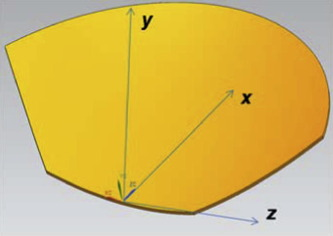Figure 2. Secondary hyperboloid reflector.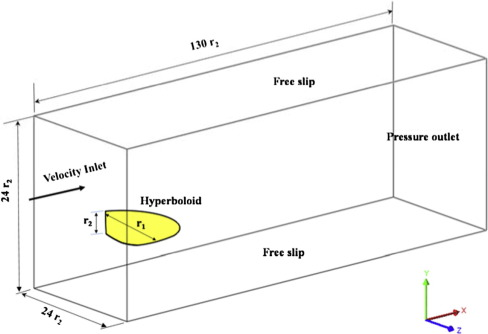Figure 3. Computational domain.

The Pressure Implicit with Splitting of Operators (PISO) is an efficient method to solve the Navier–Stokes equations in unsteady problems. The PISO algorithm  is used to deal with the pressure–velocity coupling between the momentum and the continuity equations. The spatial schemes for interpolation, gradient, Laplacian and divergence are linear, Gauss linear, Gauss linear corrected and Gauss linear schemes, respectively with second order accuracy. The cell-center values of the variables are interpolated at face locations using the second-order central difference scheme for the diffusive terms. The convection terms are discretized by a second-order accurate bounded total variational diminishing (TVD) scheme while the advancement in time is accomplished by the second-order accurate Crank–Nicolson method is employed to advance the terms in time, with the exception of the pressure term which is treated fully implicitly, i.e. it was evaluated at the new time step, instead of being split into two terms, one on the old and one on the new time step.

### 2.3. Grid generation and discretization

It is expected to acquire wind loads on buildings and structures by calculating flow field around bluff bodies within flow turbulence which simulates the boundary-layer type of flow structures applicable to wind engineering. In order to create the boundary layers around the 3D reflector, flow domain is divided into several small blocks. In order to check the quality and consistency, different meshes are created and tested. Finally, flow domain is discretized with 4.2 million elements as shown in Fig. 4. As suggested by Bruno et al. , wall-resolved LES should be in between 15 and 40. In the present work, to capture the flow behavior near structure, the dimensionless first layer height, y+ is kept 3–15 in y direction while 10–23 and 15–45 in x and z directions respectively. Generated meshes are then converted into OpenFOAM format for processing. In a grid, the velocity components are calculated for the points located on the main control volume surfaces, i.e., the staggered points, while pressure, is calculated for the points located on the main grid. The time is non-dimensionalized by U and L. The non-dimensional time-step is set equal to Δt = 1E − 4, which provides an accurate advancement in time and a CFL number close to unit. Computations are carried out on 8 Intel Quadcore X5355 2.66 GHz CPUs and require about 2.5 GB of RAM memory and 15 days of CPU time for the whole simulation.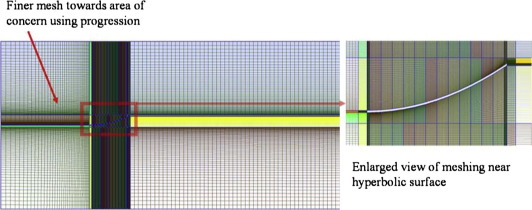Figure 4. Structured with boundary layers mesh.

## 3. Results and discussion

The dimensionless flow parameters are defined as follows,

 $C_{f\quad X}=2F_{x}/\rho U^{2}A{\mbox{,}}\quad C_{f\quad y}=$$2F_{y}/\rho U^{2}A{\mbox{,}}\quad C_{f\quad Z}=2F_{z}/\rho U^{2}A$
(6)

where ${\textstyle F_{x}}$, ${\textstyle F_{y}}$ and ${\textstyle F_{z}}$ denote the mean forces on the reflector in x, y and z directions respectively. The ρ, A and, U denote the fluid density in kilogram per unit volume, frontal area (L × r1) in meter square and free stream flow velocity in meter per second respectively. Dimensionless flow parameters are also given by,

 $Re={\frac {\rho UL}{\mu }}{\mbox{,}}\quad St={\frac {f_{v}L}{U}}{\mbox{,}}\quad T=$${\frac {tU}{L}}$
(7)

where Re is the Reynolds number, St is the Strouhal number, T   is the dimensionless time, ${\textstyle \mu }$ is the fluid dynamic viscosity, L is the characteristic length (i.e., r2), t is the time in second at each time step and fv is the frequency of vertex shedding which can be calculated from the oscillating frequency of lift force.

### 3.1. Flow over rectangular cylinder for validation

The high Re number flow around the square cylinder has been widely investigated since the beginning of the 1990s, on the basis of some detailed experimental measurements (e.g.  and ). The two-dimensional (2D) and three dimensional (3D) features of high Re   flow (i.e. ${\textstyle Re\geqslant 1.0\times {10}^{4}}$) have been studied by means of both experimental and computational approaches, with emphasis on its dependence on the chord-to-depth ratio, e.g. in Yu and Kareem  and  and Shimada and Ishihara .

Bruno et al.  developed benchmark on a rectangular cylinder (BARC) for 3D turbulent, high Re aerodynamics with a chord-to-depth ratio equal to 5. Similar configuration has been taken for validation as follows. Fig. 5 shows the velocity contour of 3D rectangular cylinder with chord-to-depth ratio equal to 5 in titled view with ${\textstyle Re\geqslant 4.0\times {10}^{4}}$. The comparisons of various parameters for 3D rectangular cylinder with results from literatures are shown in Fig. 6. Drag and lift co-efficient for 3D rectangular cylinder have been shown in Fig 6(a). As shown in Fig. 6(b)–(d), mean drag, lift and Strouhal number are compared with Yu and Kareem , Bruno et al.  and found in good agreement. From a qualitative point of view, 3D flow structures clearly appear while the flow field 2D main features hold. The flow seems almost 2D just downstream the separation point, while the wake structure is clearly 3D. Quantitatively, the present results are less than 5% deviation with Yu and Kareem  results, while they are up to 12% difference with Bruno et al. .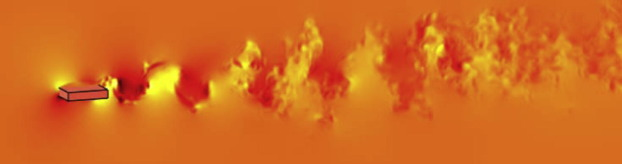Figure 5. Titled view of velocity contour slice at center of the 3D rectangular cylinder.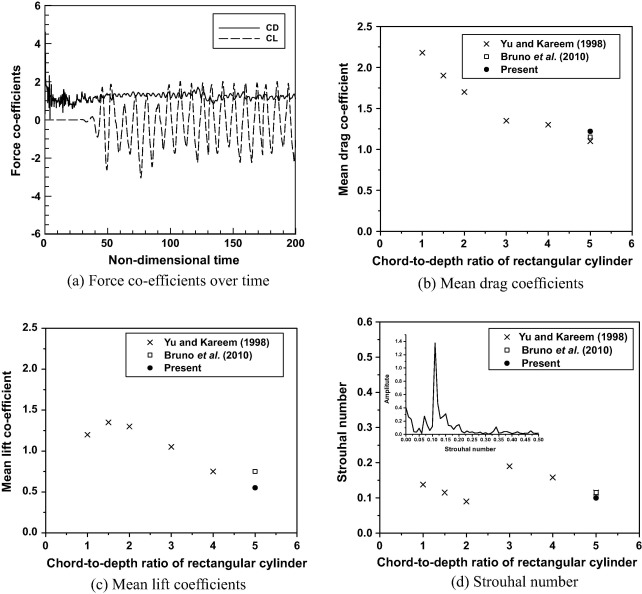Figure 6. Comparison for 3D rectangular cylinder with results from literatures.

### 3.2. Force estimation on secondary hyperboloid reflector

The total number of elements used is optimized through the grid independence study. Here, mesh size of 4.2 × 106 is taken for further analysis. In this work, the calculations are made for three wind directions and the two velocities say operational wind speed (40 km/h) and survival wind speed (160 km/h). Numerical test cases are listed in Table 1. The wind directions are taken as follows, wind flow in positive x direction (case 1), wind flow in negative x direction (case 2), wind flow in positive z direction (case 3). The x, y and z directions are in correspondence with Fig. 2. Since cases 1 and 2 computational domains are almost similar except the wind direction for case 3 the shape of the object is changed accordingly. The average and maximum force co-efficients have been tabulated in Table 2.

Table 1. Test cases conducted for hyperboloid reflector.
Case Sub-clause Wind speed Wind directiona
1 (a) Operational wind speed (40 km/h) Positive x direction
(b) Survival wind speed (160 km/h) Positive x direction
2 (a) Operational wind speed (40 km/h) Negative x direction
(b) Survival wind speed (160 km/h) Negative x direction
3 (a) Operational wind speed (40 km/h) Positive z direction
(b) Survival wind speed (160 km/h) Positive z direction

a. The x, y and z directions are depicted in Fig. 2.

Table 2. Force coefficients and Strouhal numbers.
Sl. No Operational wind speed (40 km/h) Survival wind speed (160 km/h)
Cfx Cfy Cfz St Cfx Cfy Cfz St
Case 1 1.03 −4.2 0.0002 0.4 0.625 −3.64 0.001 0.1615
Case 2 0.728 2.18 0.0002 0.32 0.4818 1.28 0.001 0.14158
Case 3 0.301 −0.943 0.0004 0.028 0.302 -0.612 0.0024 0.019

The force co-efficients for case 1(a) are shown in Fig. 7. The x, y and z average force coefficients for case 1(a) are 1.0362, 4.2 and 0.0002 respectively. Since the lift force in upward direction, the average value is in negative directions. This is caused due to the shape of the bluff body against wind load. Similarly the force coefficients are found for the other cases. The path line-profile and vorticity profile are shown for case 1(a) through 3(a) in Figure 8 and Figure 9. They are visualizing the wind turbulence at wake region. In this study, tip shedding is observed at center and edges of bluff body. The tip flow can interact with the regular Kármán vortex shedding, which may suppress the vortex shedding process (for small-aspect-ratio cylinders) or even change the vortex shedding frequency . At sharp edges in corners of the dish, the tip shedding is found. This shedding is captured and shown in Fig. 10. The frequency is found for the same through Fast Fourier Transformation (FFT) analysis. Strouhal number for the tip shedding is 1.2 as depicted in Fig. 11. Since FFT is giving two frequencies, it is good practice to check the dominant frequency over time. For this wavelet analysis is performed. Fourier transformation is useful only for a stationary signal where the amplitude-frequency does not change with time . But, in real life cases the signals are transient and also non-stationary.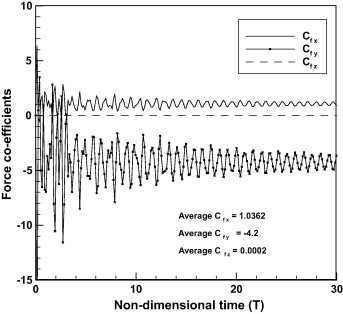Figure 7. Force co-efficient fluctuation in x, y and z directions due to tip shedding at operational wind speed for case 1(a).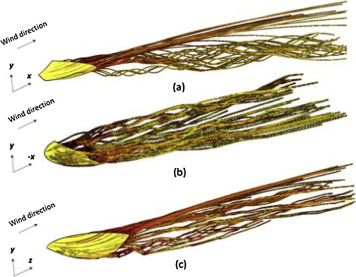Figure 8. Pathline profile of vorticity in a flow field (a) case 1(a), (b) case 2(a), and (c) case 3(a).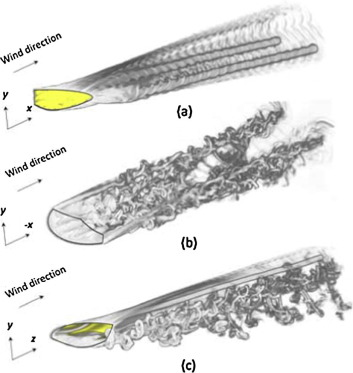Figure 9. Vorticity iso-surface field in wake region (a) case 1(a), (b) case 2(a), and (c) case 3(a).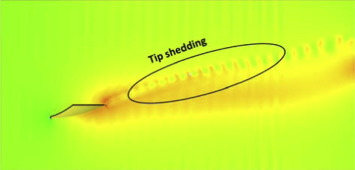Figure 10. Velocity contour for tip shedding at operational wind speed for case 1(a).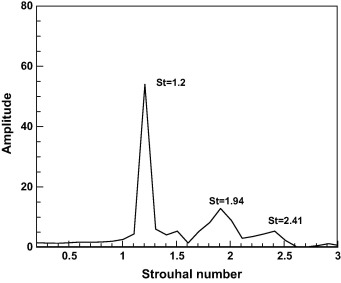Figure 11. Tip shedding frequency at operational wind speed for case 1(a).

Fig. 12 shows the wavelet analysis of tip frequency data. Real coefficient contour from the wavelet analysis shows the frequency details over time. Up to 3 s, St = 1.94 is also found along with the dominant frequency. However, after 3 s, single dominant frequency is found and St = 1.2 which is not much varying with respect to time.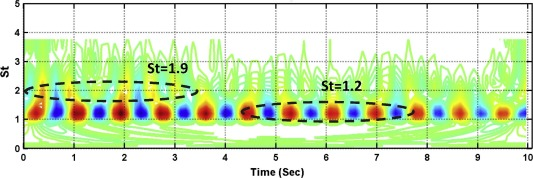Figure 12. Frequency–time analysis of tip shedding flow for case 1(a).

Fig. 13 shows the vortex formation snapshots (case 1) around the hyperboloid. The patterns are sampled with a step equal to ∼1.1 non-dimensional time unit during a sampling windows equal to ∼3 non-dimensional time units, corresponding to approximately half a period of the fluctuating lift force (i.e., 4.08 Hz). Some known clockwise and counter clockwise vortices are sketched. Dashed lines joint the vortex center in successive times. The sequence mainly takes into test the shedding development of primary and secondary vortices and the related flow structures. This qualitative examination of the sequence does not target to provide the rigorous measure of the flow structures, but to contribute to understanding the essential physics of the local instantaneous flow (see Fig. 14).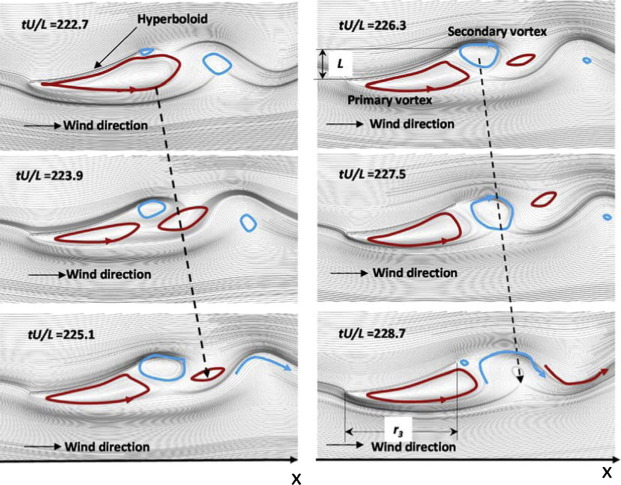Figure 13. Vortex formations snapshots (case 1) around the hyperboloid.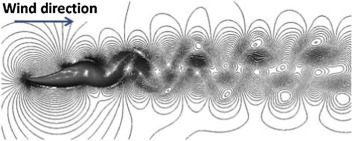Figure 14. Velocity contour for case 1(a) during operational wind speed and ${\textstyle tU/L=250}$.

### 3.3. Frequency estimation on secondary hyperboloid reflector

Strouhal number is a significant feature of fluid which has a strong dependence on both Reynolds number and spacing. Vortex shedding is an oscillating flow that takes place when a fluid flows past a bluff body at certain velocities, and it depends on the size and shape of the body and Reynolds number of fluid flow. If the structure is not mounted rigidly and vortex shedding frequency matches with the resonance frequency of the structure, the structure can start to resonate, vibrating with harmonic oscillations driven by the energy of the flow. To capture the shedding behavior and reduce the computational effort, 2D analysis is also performed to estimate shedding frequency and pressure contours.

#### 3.3.1. Wind flow in positive x direction (case 1)

In this case, operational and survival wind velocities are taken to find shedding frequency. Velocity and pressure contours and streamline diagram at operational wind speed are depicted in Figure 16 and Figure 17. The velocity and pressure fluctuations in the wake region create an oscillating flow in rear of the structure. Drag is generated by the difference in velocity between the solid object and the fluid. Fig 16 shows the velocity magnitude contour and coefficient of pressure is shown in pressure contour as shown in Fig 15. First and higher mode frequencies are found and depicted in Fig. 16. It is found that the one primary and one secondary vortex are formed behind the structure for all the cases as shown in Fig. 17. The shedding frequency has been calculated from lift force fluctuations. The velocity at x and y components and pressure contour are shown in Figure 18, Figure 19, Figure 20 and Figure 21. For case 1, the region above the structure has a positive pressure, while the just behind the structure holds the negative u velocity. Alternate convective shedding rolls have been observed from u and v contours. The velocity values are depicted in the respective place in the picture itself.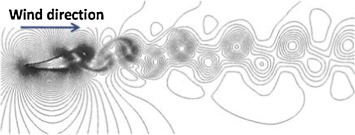Figure 15. Pressure contour for case 1(a) during operational wind speed at ${\textstyle tU/L=250}$.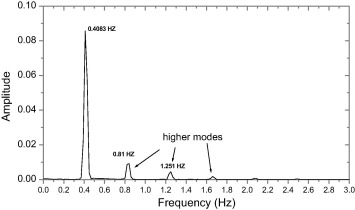Figure 16. FFT analysis for case 1(a) during operational wind speed at ${\textstyle tU/L=250}$.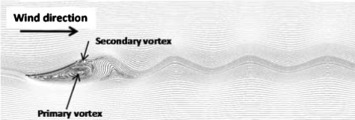Figure 17. Streamline diagram for case 1(a) during operational wind speed at ${\textstyle tU/L=250}$.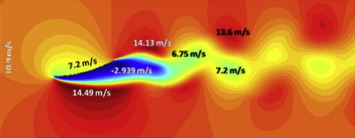Figure 18. Velocity at x component (u  ) contour for case 1(a) during operational wind speed at ${\textstyle tU/L=250}$.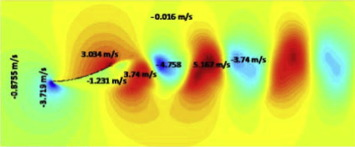Figure 19. Velocity at y component (v  ) contour for case 1(a) during operational wind speed at ${\textstyle tU/L=250}$.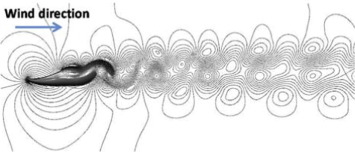Figure 20. Velocity contour for case 1(b) during survival wind speed and ${\textstyle tU/L=250}$.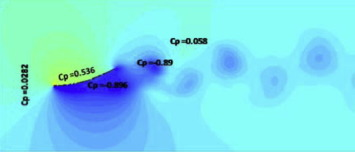Figure 21. Pressure contour for case 1(b) during survival wind speed at ${\textstyle tU/L=250}$.

Wind speeds are varied between operational and survival wind speeds, and Strouhal number has been calculated and illustrated as dimensional form in Fig. 22. Drag and lift forces are increasing while increasing the wind velocity. However, the lift force is increasing sharply compared to drag force. The structural first mode frequency has been calculated as 1.02 Hz, as shown in Fig. 22 and the wind velocity corresponds to structure frequency is 100 km/h. Hence, the plant can be operated up to 81 km/h after including safety margin of 25% with structure frequency. If the wind shedding frequency matches with tower structural frequency (i.e., 1.02 Hz), the structure may start to resonate, vibrating with harmonic oscillations driven by the energy of the flow and subsequently, deflection will be increased in the tower.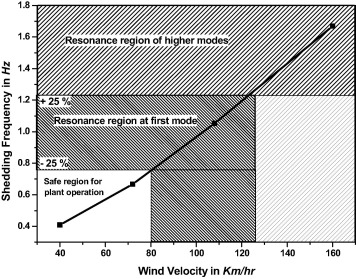Figure 22. Shedding frequency with wind velocity for case 1.

#### 3.3.2. Wind flow in negative x direction (case 2)

In this analysis, the wind direction is considered in negative x direction as shown in Fig. 2. The streamline plots for case 2 at operational and survival wind speeds are shown at T = 250 in Figure 23 and Figure 24. These streamline plots show the primary and secondary vortices. Different recirculation regions can be found on the leeward side of the hyperboloid reflector. The shape of the primary vortex is slightly more in the survival wind speed. Compared to operational speed, the secondary vortex size is also more fluctuating with respect to time at survival wind speed. The force coefficients and Strouhal number are shown in Table 2.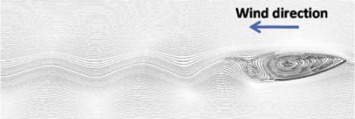Figure 23. Streamline plot at operational wind speed and ${\textstyle tU/L=250}$ (case 2(a)).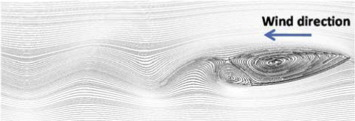Figure 24. Streamline plot at survival wind speed at ${\textstyle tU/L=250}$ (case 2(b)).

#### 3.3.3. Wind flow in positive z direction (case 3)

While comparing to the previous cases, in the case 3 computational domain and shape of the object are dissimilar. Here the 2-D cut section is taken from the yz coordinate of hyperboloid, since wind directions are similar in both directions, i.e., positive and negative z directions. The drag, lift coefficients and Strouhal number for this case are shown in Table 2. Strouhal number is calculated from the first mode frequency. Since the shape of the reflector guides the wind flow around structure, it holds low pressure coefficient around the reflector. Hence, case 3 shedding frequency is relatively low. The wind force on the reflector increases sharply while the wind speed increases. When the flow direction is in positive x direction (case 1), then the lift force is negative. That means the lift force on structure is acting toward ground. Flow direction is in negative x direction (case 2), and now situation is just opposite. That is, the lift force is acting opposite to gravity. Using the force coefficient, total wind load on secondary reflector has been calculated for three cases, while the same is applied on the reflector tower to check the structural integrity in the following section.

## 4. Reflector tower analysis

Hyperboloid reflector has been designed as a truss structure as per Indian Standard Code of Practice for General Construction in Steel (IS: 800). Structural tubes of different sizes have been used as truss members to reduce deflections under dead weight and wind loads. Hollow sections have been chosen as truss members as it is inherently strong, efficient and gives a better strength-to-weight ratio. Fig. 25 shows the top view of the hyperbolic reflector. Hyperboloid has a ring beam as shown in Fig. 26. It is of 1.2 m depth and 1.0 m width. Hyperboloid structure has two layers of trusses connected by vertical and diagonal members as shown in Fig. 27. The distance between the two planes of trusses has been kept 1.2 m as aluminum roll of 1.2 m width is available. Depth of truss has been optimized and is 0.6 m. This hyperboloid is supported on three towers. Towers have been designed to ensure fundamental frequency beyond 1 Hz. Pre-stressed cables have been used to limit the deflections in hyperboloid reflecting surface. Finite element analysis has been performed to optimize the design and estimate the deflections and stresses due to dead weight, imposed loads and wind loads. Modal analysis has also been performed to find out natural frequencies. In finite element model beam elements have been used to model the structural members. Lumped mass elements have been used to model imposed loads. Link elements have been used to model cables used for controlling deflection in hyperboloid reflecting surface. Fig. 28 shows the finite element model of hyperboloid and central tower.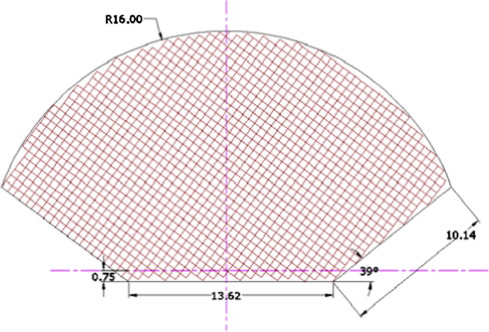Figure 25. Hyperbolic reflector (plan).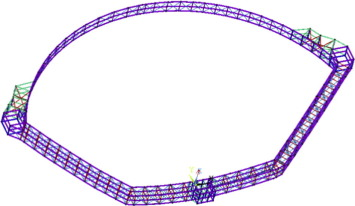Figure 26. Ring beam.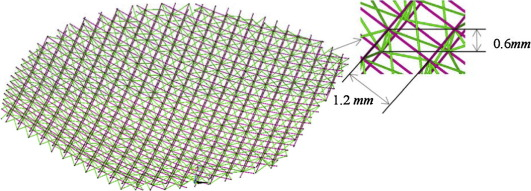Figure 27. Layer of trusses.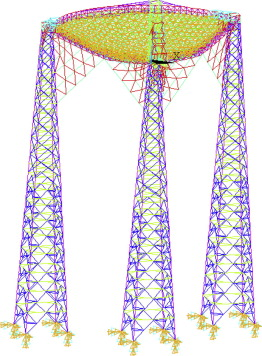Figure 28. Finite element model of hyperboloid and central tower.

### 4.1. Static and modal analysis

Static analysis has been performed to calculate the deflections and stresses due to dead weight and imposed loads. Total mass of the structure is 58,630 kg. 8000 kg imposed loads (dead weight of aluminum sheet and supporting structure) have been considered. Fig. 29 shows the deformed shape due to dead weight without pre-stressed cables. Since the deflection is very high, pre-stressed cable has been used to reduce the deflections in hyperboloid structure. Fig. 30 shows the deformed shape due to dead weight of secondary hyperboloid and central tower with pre-stressed cables. Modal analysis has been performed to obtain the natural frequencies, mode shapes and participation factors in each mode. Some of the dominant modes have been listed in Table 3. Figure 31 and Figure 32 show first and second mode shapes.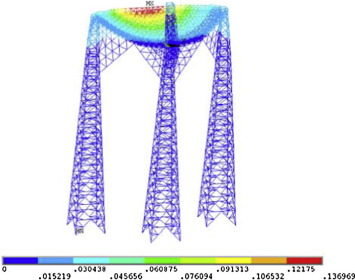Figure 29. Deformed shape without pre-stressed cables (dead load).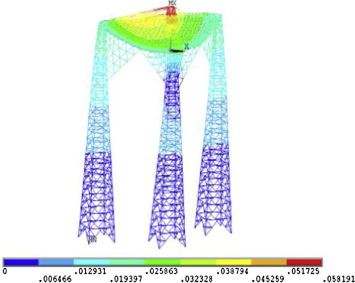Figure 30. Deformed shape with pre-stressed cables (dead load).

Table 3. Frequencies and participation factors.
S. No. Frequency (Hz) X-direction Y-direction Z-direction
MPF  %MP MPF  %MP MPF  %MP
1. 1.02 185.73 51.77 0.595 −0.137
2. 1.06 −0.315 217.40 70.93 −24.02 0.87
3. 1.73 132.11 26.19 0.768 2.60 0.01
4. 1.97 −2.89 57.24 4.92 113.71 19.41

MPF – Mass Participation Factors; %MP – Percentage Mass Participation.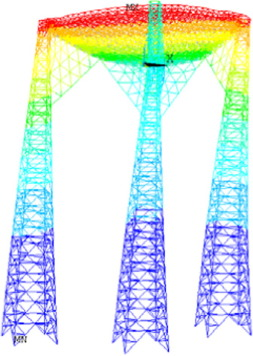Figure 31. First mode shape (1.02 Hz).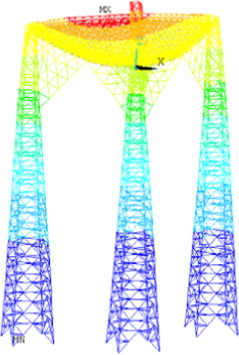Figure 32. Second mode shape (1.06 Hz).

Since IS 875 Part 3 does not provide force coefficient for hyperboloid surface, CFD analysis has been performed to find out the forces due to wind loads on hyperboloid surface. Analysis has been performed for three different cases to estimate the deflections and stresses in hyperboloid and tower due to dead weight, imposed loads and wind loads. In cases 1, 2 and 3 wind loads have been considered in X, −X and Z directions respectively. Deflections in hyperboloid and tower are estimated for operational and survival wind and tabulated in Table 4. Stresses due to various loads and their combinations have been estimated and compared against the allowable stresses as per IS 800  and are found within the allowable limit ( Figure 33 and Figure 34). Maximum stresses due to dead loads, imposed loads and wind loads (survival) have been tabulated in Table 4.

Maximum deflections in (mm) Axial tension stress at survival wind speed (MPa) Axial compression stress at survival wind speed (MPa) Bending stress at survival wind speed (MPa)
Operational wind speed Survival wind speed
Case 1 8.69 139.0 119 143 180
Case 2 8.21 131.3 55 89 117
Case 3 5.86 93.73 118 146 185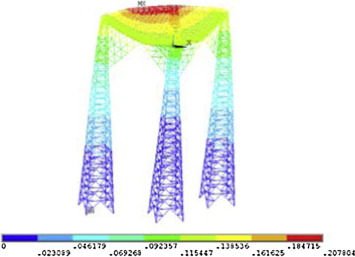Figure 33. Deformed shape of central tower due to survival wind load for case 1. (Dead loads + imposed loads + wind loads.)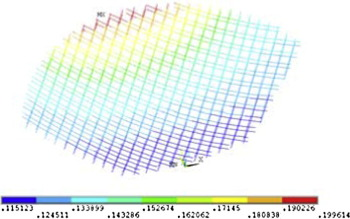Figure 34. Deformed shape of support frames of reflector due to wind load for case 1. (Dead loads + imposed loads + wind loads.)

## 5. Conclusions

The wind load on solar power plant secondary reflector is estimated through LES simulation. Force coefficients are evaluated for different wind direction cases under operational (40 km/h) and survival (60 km/h) wind speeds. Since IS 875 Part 3 does not provide force coefficient for hyperboloid surface, CFD analysis has been performed to find out the forces due to wind loads on hyperboloid surface. Shedding frequencies have also been obtained by CFD analysis. The wind directions are also varied into three cases, viz., wind flow in positive x direction (case 1), wind flow in negative x direction (case 2), and wind flow in positive z direction (case 3). Here, the forces and vortex shedding frequency are increasing while increasing the wind velocity. Finite element analysis has been performed to find out the structural natural frequencies, deflections and stresses due to wind load along with dead and imposed loads. From aforesaid work, the following conclusions are drawn.

• Fundamental frequency of the structure is 1.02 Hz, which is quite away from shedding frequency estimated by CFD analysis. Also IS 800  recommends frequency more than 1.0 Hz to avoid wind oscillation. If the wind shedding frequency matches with tower structural frequency (i.e., 1.0 Hz), the structure can start to resonate, vibrating with harmonic oscillations driven by the energy of the flow and subsequently, deflection will be increased in the tower.
• From Fig. 22, it can be observed that the wind velocity corresponds to structural fundamental frequency 1.02 Hz is 105 km/h, considering resonance frequency band of ±25% the wind velocity up to which plant can be operated is ∼80 km/h.
• Drag and lift forces are increasing while increasing the wind velocity. However, the lift force is increasing sharply compared to drag force. When the flow direction is in positive x direction (case 1), then the lift force is negative. That means the lift force on structure is acting toward ground. When flow direction is in negative x direction (case 2), the lift force is acting opposite to gravity.

The tip flow can interact with the regular Kármán vortex shedding, which may suppress the vortex shedding process or even change the vortex shedding frequency. At sharp edges in corners of the dish, the tip shedding is found. It is found from wavelet analysis of tip shedding flow the first three seconds due to initial turbulence, an additional frequency (St = 1.94) along with the dominant frequency. However, after 3 s, single dominant frequency is found and St = 1.2 which is not much varying with respect to time.

• The shape of the reflector guides the wind flow around structure while for wind flowing from positive z direction, it holds low pressure coefficient around the reflector resulting relatively low shedding frequency.
• Real coefficients contour from the wavelet analysis shows the frequency details over time. Up to 3 s, St = 1.94 is also found along with the dominant frequency. However, after 3 s, single dominant frequency is found and St = 1.2 which is not much varying with respect to time.
• To reduce the tower oscillation, pre-stressed cables are used. Maximum deformation on tower is found as 13.6 mm and 5.6 mm for without and with pre-stressed cables respectively.
• The calculated stresses are found within acceptable limit for both operational and survival wind speeds. This study can be extended by finding the suitable drag minimization techniques to avoid the resonance matching.

### Document informationPublished on 12/04/17

Licence: Other

### Document Score0

Views 42
Recommendations 0